# Chi-squared test

(diff) ← Older revision | Latest revision (diff) | Newer revision → (diff)

A test for the verification of a hypothesisaccording to which a random vector of frequencies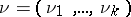has a given polynomial distribution, characterized by a vector of positive probabilities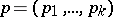,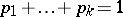. The "chi-squared" test is based on the Pearson statisticwhich has in the limit, as, a "chi-squared" distribution withdegrees of freedom, that is,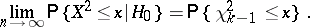According to the "chi-squared" test with significance level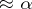, the hypothesis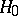must be rejected if, where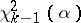is the upper-quantile of the "chi-squared" distribution with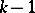degrees of freedom, that is,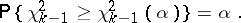The statistic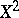is also used to verify the hypothesisthat the distribution functions of independent identically-distributed random variables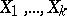belong to a family of continuous functions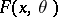,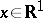,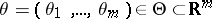,an open set. After dividing the real line by points,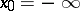,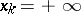, intointervals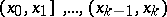,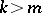, such that for all,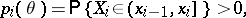;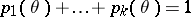, one forms the frequency vector, which is obtained as a result of grouping the values of the random variables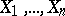into these intervals. Letbe a random variable depending on the unknown parameter. To verify the hypothesisone uses the statistic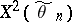, where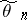is an estimator of the parameter, computed by the method of the minimum of "chi-squared" , that is,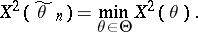If the intervals of the grouping are chosen so that all, if the functions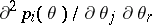are continuous for all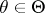,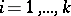;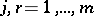, and if the matrix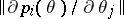has rank, then if the hypothesisis valid and as, the statistichas in the limit a "chi-squared" distribution withdegrees of freedom, which can be used to verifyby the "chi-squared" test. If one substitutes a maximum-likelihood estimator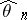in, computed from the non-grouped data, then under the validity ofand as, the statistic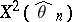is distributed in the limit like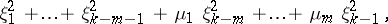where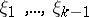are independent standard normally-distributed random variables, and the numbers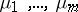lie between 0 and 1 and, generally speaking, depend upon the unknown parameter. From this it follows that the use of maximum-likelihood estimators in applications of the "chi-squared" test for the verification of the hypothesisleads to difficulties connected with the computation of a non-standard limit distribution.

In  there are some recommendations concerning the-test in this case; in particular, in the normal case , the general continuous case , , the discrete case , , and in the problem of several samples .

How to Cite This Entry:
Chi-squared test. Encyclopedia of Mathematics. URL: http://encyclopediaofmath.org/index.php?title=Chi-squared_test&oldid=15852
This article was adapted from an original article by M.S. Nikulin (originator), which appeared in Encyclopedia of Mathematics - ISBN 1402006098. See original article